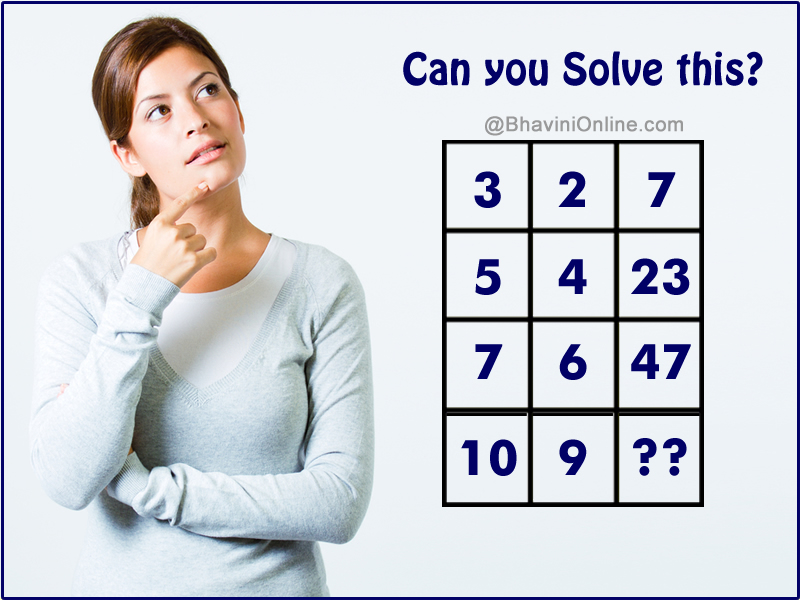# Fun Maths Riddle: If 3 | 2 = 7; Then 10 | 9 = ??

Look at the numbers in the table and find the missing number in the riddle.

You will have to crack the logic and the relationshop between the number to find the missing number.3 2 7 5 4 23 7 6 47 9 8 79 10 9 ??

The logic followed in the given equations are as follows;

R1 x R2 + (R2 – 1) = R3

So if we apply this in the given table, you will see;

3 x 2 + (2 – 1) = 6 + 1 = 7

Similarly for other rows we can solve the equation as;

5 x 4 + (4 – 1) = 20 + 3 = 23

7 x 6 + (6 – 1) = 42 + 5 = 47

9 x 8 + (8 – 1) = 72 + 7 = 79

Therefore we can find the missing number as;

10 x 9 + (9 – 1) = 90 + 8 = 98

## 3 Replies to “Fun Maths Riddle: If 3 | 2 = 7; Then 10 | 9 = ??”

1. chitesh kumar says:

97, r1+r2+1, r1+r2+3, r1+r2+5, r1+r2+7=97

2. Maryam says:

Please explain me how n why did we apply this multiplication and subtraction in between to get the answer. I mean how do we know what to n how to use where n in which equation. Specially for this 3 2=7 and 10 9=

3. Ahmed Skeptic says:

I can argue that :

Let’s take U(0) = -1 , and U(n+1) = U(n) + 2

Then R1xR2+U(n+1) explains all above but changes the result of the answer to 97.